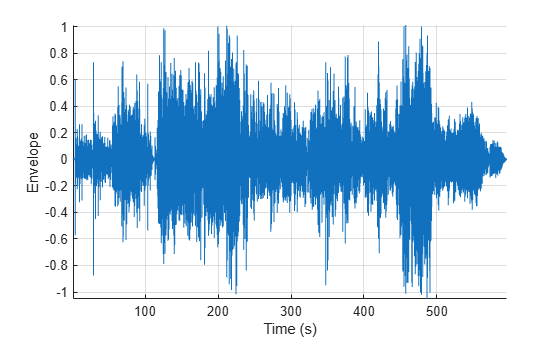# audioEnvelope

Compute envelope of an audio file

Since R2023a

## Syntax

``[minEnv,maxEnv] = audioEnvelope(audioIn)``
``[minEnv,maxEnv] = audioEnvelope(filename)``
``[minEnv,maxEnv] = audioEnvelope(___,Name=Value)``
``[minEnv,maxEnv,loc] = audioEnvelope(___)``
``[minEnv,maxEnv,loc,fs] = audioEnvelope(___)``
``audioEnvelope(___)``

## Description

example

````[minEnv,maxEnv] = audioEnvelope(audioIn)` returns the envelope of the input audio signal. The audio envelope contains maximum and minimum values over nonoverlapping frames of the input signal, and it approximates the shape of the waveform.```

example

````[minEnv,maxEnv] = audioEnvelope(filename)` returns the envelope of the audio file.```

example

````[minEnv,maxEnv] = audioEnvelope(___,Name=Value)` specifies options using one or more name-value arguments. For example, `audioEnvelope(filename,Range=[1000, 5000])` computes the envelope of the signal from samples 1000 through 5000 in the audio file.```

example

````[minEnv,maxEnv,loc] = audioEnvelope(___)` also returns the locations of the envelope frames within the original signal.```

example

````[minEnv,maxEnv,loc,fs] = audioEnvelope(___)` also returns the sample rate of the audio signal.```

example

````audioEnvelope(___)` with no output arguments plots the audio envelope.```

## Examples

collapse all

Compute the envelope of a file containing a 10-minute audio signal.

`[envMin,envMax] = audioEnvelope("SoftGuitar-44p1_mono-10mins.ogg");`

Plot the envelope by calling `audioEnvelope` with no output arguments.

`audioEnvelope("SoftGuitar-44p1_mono-10mins.ogg")`Read in a 10-minute audio signal from a file.

`[audioIn,fs] = audioread("SoftGuitar-44p1_mono-10mins.ogg");`

Compute the audio envelope of the signal.

`[envMin,envMax] = audioEnvelope(audioIn);`

Call `audioEnvelope` with no output arguments to plot the envelope.

`audioEnvelope(audioIn)`Specify the `SampleRate` of the signal to see time on the x-axis of the plot instead of samples.

`audioEnvelope(audioIn,SampleRate=fs)`Compute the envelope from samples 5000 to 10,000 of the audio file. Set the number of points in the envelope to be 500.

```[envMin,envMax] = audioEnvelope("Counting-16-44p1-mono-15secs.wav", ... Range=[5000, 10000],NumPoints=500);```

Plot the envelope of the specified size and range.

```audioEnvelope("Counting-16-44p1-mono-15secs.wav", ... Range=[5000, 10000],NumPoints=500);```Compute the envelope of an audio file. Specify additional output arguments to obtain the locations of the envelope frames and the sample rate of the audio signal.

`[minEnv,maxEnv,loc,fs] = audioEnvelope("SoftGuitar-44p1_mono-10mins.ogg");`

Use the sample rate to convert the frame locations from samples to seconds.

`loc = loc./fs;`

Plot the maximum and minimum values of the envelope with the time of the original audio signal on the x-axis.

```plot(loc,maxEnv,loc,minEnv) xlabel("Time (s)")```## Input Arguments

collapse all

Audio input signal, specified as a column vector or matrix. If the input is a matrix, the columns are treated as individual channels.

Data Types: `single` | `double`

Name of the audio file, specified as a string scalar or character vector. `audioEnvelope` accepts the same file formats as `audioread`.

Data Types: `char` | `string`

### Name-Value Arguments

Specify optional pairs of arguments as `Name1=Value1,...,NameN=ValueN`, where `Name` is the argument name and `Value` is the corresponding value. Name-value arguments must appear after other arguments, but the order of the pairs does not matter.

Example: `audioEnvelope(audioIn,NumPoints=5000)`

Number of points in the audio envelope, specified as a positive integer.

Data Types: `single` | `double` | `int8` | `int16` | `int32` | `int64` | `uint8` | `uint16` | `uint32` | `uint64`

Range of the envelope in samples, specified as a row vector of two positive integers. The range specifies the start and end indices into the input signal that define the region over which to compute the envelope. The default range is the entire input signal.

Example: Range=[1000, 2000]

Data Types: `single` | `double` | `int8` | `int16` | `int32` | `int64` | `uint8` | `uint16` | `uint32` | `uint64`

Sample rate in Hz, specified as a positive scalar.

You can use this name-value argument only when the input is a numeric array. If the input is a file name, the function derives the sample rate from the audio file information.

Data Types: `single` | `double`

## Output Arguments

collapse all

Minimum values of the envelope, returned as a `NumPoints`-by-C matrix, where C is the number of channels in the input signal. The values are the minimums over nonoverlapping frames in the input signal. The frame size is equal to `floor(L/NumPoints)`, where L is the length of the signal.

Maximum values of the envelope, returned as a `NumPoints`-by-C matrix, where C is the number of channels in the input signal. The values are the maximums over nonoverlapping frames in the input signal. The frame size is equal to `floor(L/NumPoints)`, where L is the length of the signal.

Frame locations, returned as a row vector of length `NumPoints`. The locations are indices into the input signal of the most recent sample of each frame.

Sample rate of the input signal in Hz, returned as a positive scalar.

If you specify the input signal as a numeric array instead of a file and do not specify the `SampleRate` argument, then the sample rate is returned as 1.

## Version History

Introduced in R2023a